# simulate

Monte Carlo simulation of ARIMA or ARIMAX models

## Syntax

```[Y,E] = simulate(Mdl,numObs) [Y,E,V] = simulate(Mdl,numObs) [Y,E,V] = simulate(Mdl,numObs,Name,Value) ```

## Description

```[Y,E] = simulate(Mdl,numObs)``` simulates sample paths and innovations from the ARIMA model, `Mdl`. The responses can include the effects of seasonality.

```[Y,E,V] = simulate(Mdl,numObs)``` additionally simulates conditional variances, `V`.

`[Y,E,V] = simulate(Mdl,numObs,Name,Value)` simulates sample paths with additional options specified by one or more `Name,Value` pair arguments.

## Input Arguments

 `Mdl` ARIMA or ARIMAX model, specified as an `arima` model returned by `arima` or `estimate`. The properties of `Mdl` cannot contain `NaN`s. `numObs` Positive integer that indicates the number of observations (rows) to generate for each path of the outputs `Y`, `E`, and `V`.

### Name-Value Pair Arguments

Specify optional comma-separated pairs of `Name,Value` arguments. `Name` is the argument name and `Value` is the corresponding value. `Name` must appear inside quotes. You can specify several name and value pair arguments in any order as `Name1,Value1,...,NameN,ValueN`.

 `'E0'` Mean zero presample innovations that provide initial values for the model. `E0` is a column vector or a matrix with at least `NumPaths` columns and enough rows to initialize the model and any conditional variance model. The number of observations required is at least `Mdl.Q`, but can be more if you specify a conditional variance model. If the number of rows exceeds the number necessary, then `simulate` only uses the most recent observations. If the number of columns exceeds `NumPaths`, then `simulate` only uses the first `NumPaths` columns. If `E0` is a column vector, then it is applied to each simulated path. The last row contains the most recent presample observation. Default: `simulate` sets the necessary presample observations to 0. `'NumPaths'` Positive integer that indicates the number of sample paths (columns) to generate. Default: `1` `'V0'` Positive presample conditional variances which provide initial values for any conditional variance model. If the variance of the model is constant, then `V0` is unnecessary. `V0` is a column vector or a matrix with at least `NumPaths` columns and enough rows to initialize the variance model. If the number of rows exceeds the number necessary, then `simulate` only uses the most recent observations. If the number of columns exceeds `NumPaths`, then `simulate` only uses the first `NumPaths` columns. If `V0` is a column vector, then `simulate` applies it to each simulated path. The last row contains the most recent observation. Default: `simulate` sets the necessary presample observations to the unconditional variance of the conditional variance process. `'X'` Matrix of predictor data with length `Mdl.Beta` columns of separate series. The number of observations (rows) of `X` must equal or exceed `numObs`. If the number of observations of `X` exceeds `numObs`, then `simulate` only uses the most recent observations. `simulate` applies the entire matrix `X` to each simulated response series. The last row contains the most recent observation. Default: `simulate` does not use a regression component regardless of the value of `Mdl.Beta`. `'Y0'` Presample response data that provides initial values for the model. `Y0` is a column vector or a matrix with at least `Mdl.P` rows and `NumPaths` columns. If the number of rows exceeds `Mdl.P`, then `simulate` only uses the most recent `Mdl.P` observations. If the number of columns exceeds `NumPaths`, then `simulate` only uses the first `NumPaths` columns. If `Y0` is a column vector, then it is applied to each simulated path. The last row contains the most recent presample observation. Default: `simulate` sets the necessary presample observations to the unconditional mean if the AR process is stable, or to 0 for unstable processes or when you specify `X`.

Notes

• `NaN`s indicate missing values, and `simulate` removes them. The software merges the presample data, then uses list-wise deletion to remove any `NaN`s in the presample data matrix or `X`. That is, `simulate` sets `PreSample` = `[Y0 E0 V0]`, then it removes any row in `PreSample` or `X` that contains at least one `NaN`.

• The removal of `NaN`s in the main data reduces the effective sample size. Such removal can also create irregular time series.

• `simulate` assumes that you synchronize the predictor series such that the most recent observations occur simultaneously. The software also assumes that you synchronize the presample series similarly.

## Output Arguments

 `Y` `numObs`-by-`NumPaths` matrix of simulated response data. `E` `numObs`-by-`NumPaths` matrix of simulated mean zero innovations. `V` `numObs`-by-`NumPaths` matrix of simulated conditional variances of the innovations in `E`.

## Examples

expand all

Simulate response and innovation paths from a multiplicative seasonal model.

Specify the model

`$\left(1-L\right)\left(1-{L}^{12}\right){y}_{t}=\left(1-0.5L\right)\left(1+0.3{L}^{12}\right){\epsilon }_{t},$`

where ${\epsilon }_{t}$ follows a Gaussian distribution with mean 0 and variance 0.1.

```Mdl = arima('MA',-0.5,'SMA',0.3,... 'SMALags',12,'D',1,'Seasonality',12,... 'Variance',0.1,'Constant',0);```

Simulate 500 paths with 100 observations each.

```rng default % For reproducibility [Y,E] = simulate(Mdl,100,'NumPaths',500); figure subplot(2,1,1); plot(Y) title('Simulated Response') subplot(2,1,2); plot(E) title('Simulated Innovations')```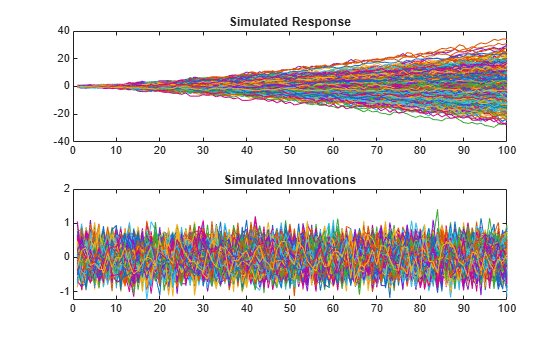Plot the 2.5th, 50th (median), and 97.5th percentiles of the simulated response paths.

```lower = prctile(Y,2.5,2); middle = median(Y,2); upper = prctile(Y,97.5,2); figure plot(1:100,lower,'r:',1:100,middle,'k',... 1:100,upper,'r:') legend('95% Interval','Median')```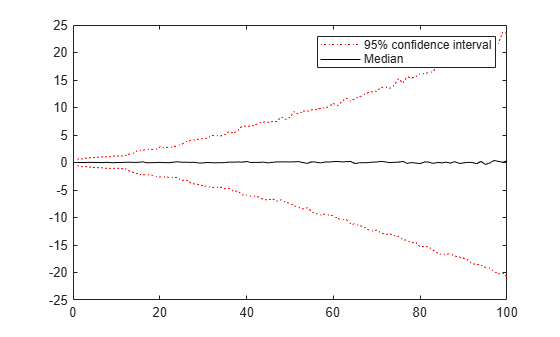Compute statistics across the second dimension (across paths) to summarize the sample paths.

Plot a histogram of the simulated paths at time 100.

```figure histogram(Y(100,:),10) title('Response Distribution at Time 100')```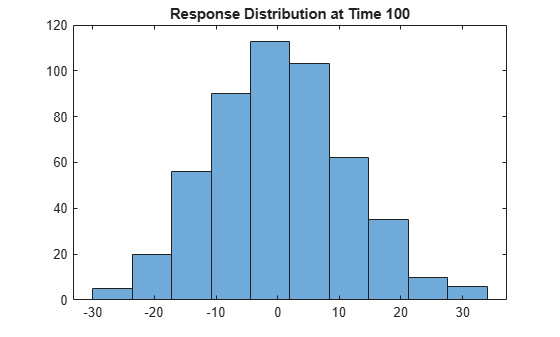Simulate three predictor series and a response series.

Specify and simulate a path of length 20 for each of the three predictor series modeled by

`$\left(1-0.2L\right){x}_{it}=2+\left(1+0.5L-0.3{L}^{2}\right){\eta }_{it},$`

where ${\eta }_{it}$ follows a Gaussian distribution with mean 0 and variance 0.01, and $i$ = {1,2,3}.

```[MdlX1,MdlX2,MdlX3] = deal(arima('AR',0.2,'MA',... {0.5,-0.3},'Constant',2,'Variance',0.01)); rng(4); % For reproducibility simX1 = simulate(MdlX1,20); simX2 = simulate(MdlX2,20); simX3 = simulate(MdlX3,20); SimX = [simX1 simX2 simX3];```

Specify and simulate a path of length 20 for the response series modeled by

`$\left(1-0.05L+0.02{L}^{2}-0.01{L}^{3}\right)\left(1-L{\right)}^{1}{y}_{t}=0.05+{x}_{t}^{\prime }\left[\begin{array}{c}0.5\\ -0.03\\ -0.7\end{array}\right]+\left(1+0.04L+0.01{L}^{2}\right){\epsilon }_{t},$`

where ${\epsilon }_{t}$ follows a Gaussian distribution with mean 0 and variance 1.

```MdlY = arima('AR',{0.05 -0.02 0.01},'MA',... {0.04,0.01},'D',1,'Constant',0.5,'Variance',1,... 'Beta',[0.5 -0.03 -0.7]); simY = simulate(MdlY,20,'X',SimX);```

Plot the series together.

```figure plot([SimX simY]) title('Simulated Series') legend('{X_1}','{X_2}','{X_3}','Y')```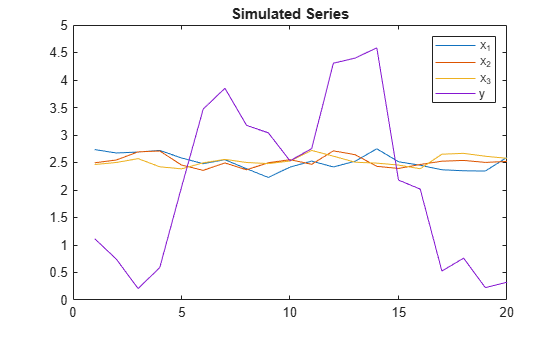Forecast the daily NASDAQ Composite Index using Monte Carlo simulations.

Load the NASDAQ data included with the toolbox. Extract the first 1500 observations for fitting.

```load Data_EquityIdx nasdaq = DataTable.NASDAQ(1:1500); n = length(nasdaq);```

Specify, and then fit an ARIMA(1,1,1) model.

```NasdaqModel = arima(1,1,1); NasdaqFit = estimate(NasdaqModel,nasdaq);```
``` ARIMA(1,1,1) Model (Gaussian Distribution): Value StandardError TStatistic PValue _________ _____________ __________ __________ Constant 0.43031 0.18555 2.3191 0.020391 AR{1} -0.074389 0.081985 -0.90735 0.36422 MA{1} 0.31126 0.077266 4.0284 5.6166e-05 Variance 27.826 0.63625 43.735 0 ```

Simulate 1000 paths with 500 observations each. Use the observed data as presample data.

```rng default; Y = simulate(NasdaqFit,500,'NumPaths',1000,'Y0',nasdaq);```

Plot the simulation mean forecast and approximate 95% forecast intervals.

```lower = prctile(Y,2.5,2); upper = prctile(Y,97.5,2); mn = mean(Y,2); figure plot(nasdaq,'Color',[.7,.7,.7]) hold on h1 = plot(n+1:n+500,lower,'r:','LineWidth',2); plot(n+1:n+500,upper,'r:','LineWidth',2) h2 = plot(n+1:n+500,mn,'k','LineWidth',2); legend([h1 h2],'95% Interval','Simulation Mean',... 'Location','NorthWest') title('NASDAQ Composite Index Forecast') hold off```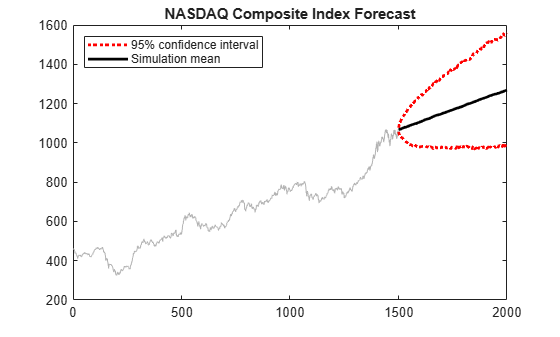## References

 Box, G. E. P., G. M. Jenkins, and G. C. Reinsel. Time Series Analysis: Forecasting and Control 3rd ed. Englewood Cliffs, NJ: Prentice Hall, 1994.

 Enders, W. Applied Econometric Time Series. Hoboken, NJ: John Wiley & Sons, 1995.

 Hamilton, J. D. Time Series Analysis. Princeton, NJ: Princeton University Press, 1994.